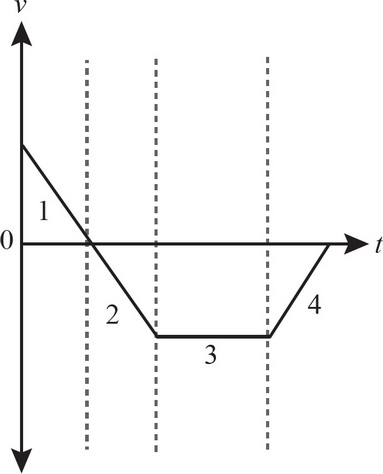# AP Physics 1 Multiple-Choice Practice Test 32

### Test Information10 questions18 minutes

1. A car traveling at 30 m/s is playing loud music with its speakers. What must the magnitude and direction of the velocity of a truck be in order for its passengers to hear the music emitted from the car with no change in the frequency?

2. As a train approaches the next stop, the conductor blows the horn and applies the brakes on the train. What will a person standing at the train stop hear as the train approaches?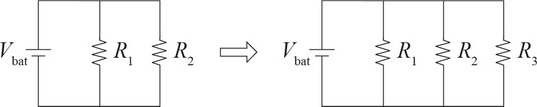3. Two resistors, R1 and R2, have equal resistance, and are connected in a circuit with a battery as shown in the figure above. If a third resistor, also with the same resistance, is then connected to the circuit, as shown, what happens to the energy E1 and E2 dissipated by R1 and R2, respectively, during a given time interval?

4. If the voltage drop across a 3 Ω resistor is 2 V, how long will it take for the resistor to dissipate 100 J of energy?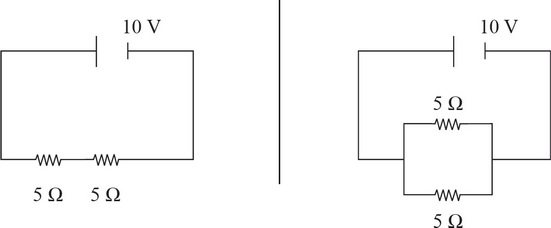5. A 10 V battery is connected to two 5 Ω resistors in series. Separately, another 10 V battery is connected to two 5 Ω resistors in parallel. What is the difference between the total current produced in each circuit?

6. Question below refers to the following scenario: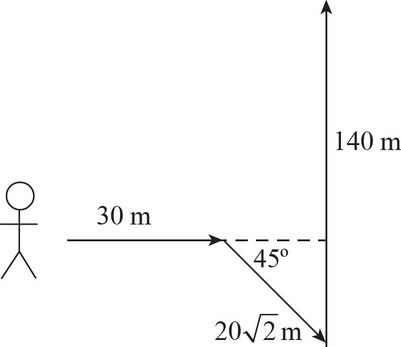An explorer travels 30 m east, then 20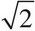m in a direction 45° south of east, and then 140 m north.

What is the distance traveled by the explorer?

7. Question below refers to the following scenario:An explorer travels 30 m east, then 20m in a direction 45° south of east, and then 140 m north.

What is the displacement of the explorer?

8. Question below refers to the following scenario:An explorer travels 30 m east, then 20m in a direction 45° south of east, and then 140 m north.

The explorer took 60 s, 130 s, and 70 s to travel the 30 m, 20m, and 140 m north distances, respectively. What is the average velocity of the explorer over the total distance traveled?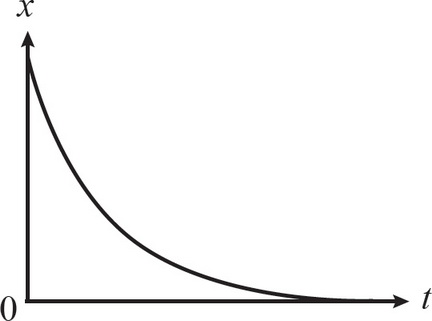9. The graph above is the position-versus-time graph of an object. Which of the following is the velocity-versus-time graph of the object?

10. In which section of the following velocity-versus-time graph is the object slowing down and moving in the negative direction?